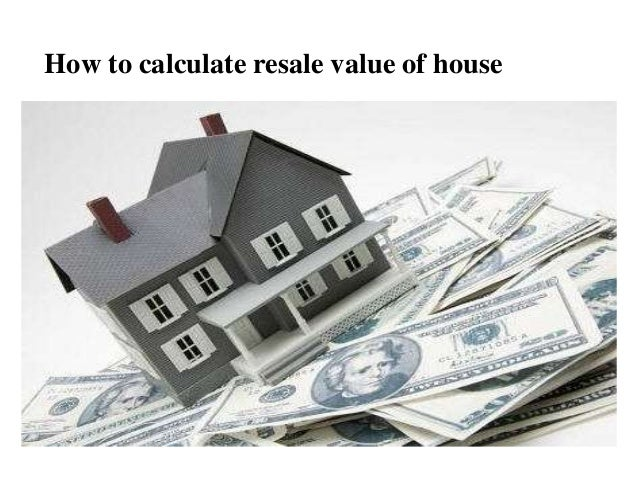Successfully reported this slideshow.
We use your LinkedIn profile and activity data to personalize ads and to show you more relevant ads. You can change your ad preferences anytime.Upcoming SlideShare
×

# How to calculate resale value of house

588 views

Published on

Planning to buy a resale property? We provide a rundown of the pros and cons on how to calculate resale value of house. Find the one that suits your needs.

Published in: Economy & Finance
• Full Name
Comment goes here.

Are you sure you want to Yes No
Your message goes here• Be the first to comment

• Be the first to like this

### How to calculate resale value of house

1. 1. How to calculate resale value of house
2. 2. How to calculate resale value of house? For a resale flat one has to consider value of land, estimated life of building, age of building and facilities available. lets take for example a 20 years flat with 1500 sq ft built up 1000 sq ft UDS. If the flat was to be constructed today assuming the market price of land is 10,000/sqft
3. 3. cost of land = (UDS * current market price) = 1,000 * 10,000 = 1,00,00,000 cost of construction = (built up area * construction cost) = (1500*1200) = 18,00,000 Total = 1,18,00,000 including all other charges. The rate of depreciation is calculated as follows The normal age of a building is considered to be 60 years. Depreciation is calculated such that at the end of 60 years the value of the building becomes zero.
4. 4. Out of the total asset value the residual or scrap or salvage value, is normally taken at 10% and can be ignored. The remaining 90% of the building cost is spread over the 60 years. hence, rate of depreciation is 1.5% per year. So if a building is 20 years old then rate of depreciation is 30%.
5. 5. i.e. the building is 70% of its initial value. The cost of the resale flat would work out as follows if assuming the cost of construction 20 years back was 250/sqft. cost of land = 1,00,00,000 cost of building = (1-(30/100)) * (1500*250) = 2,60,000 Total cost of resale flat = 1,02,60,000
6. 6. Because the flat would have been purchased at lower input cost, at cheaper land rate and due to poor maintenance, the owner may also be willing to offer at 30-40% lesser than the calculation above. If a person who holds a old flat (25-30 years+) wants to reap the benefits as per the true valuation above. The most profitable option is to go in for a joint venture along with all the residents of the building by hiring a builder or their own contractor, View Here: How to calculate resale value of house
7. 7. With the relaxation of FSI, they can get substantial amount of money in hand and possibly also retain a flat in the complex by only sharing their space with few more additional neighbors. Source: [http://buildingvaluation.blogspot.in/2014/09/how-to-calculate- resale-value-of.html#.WABy4OV97IU]